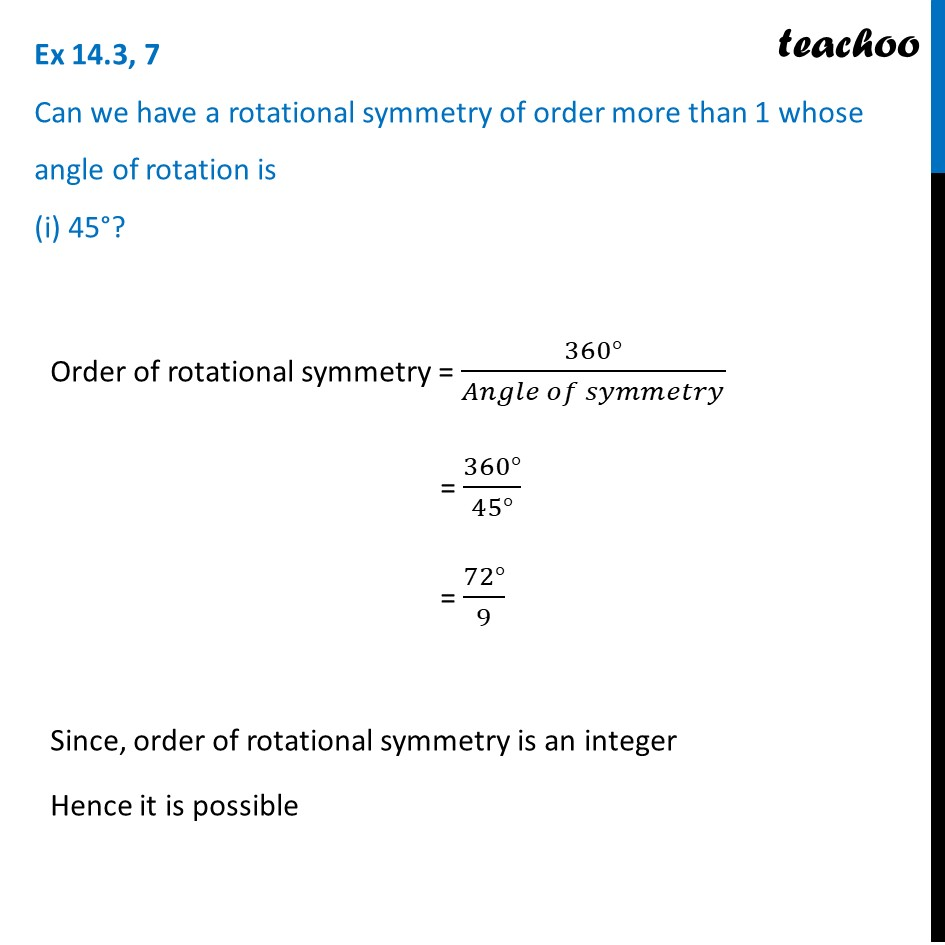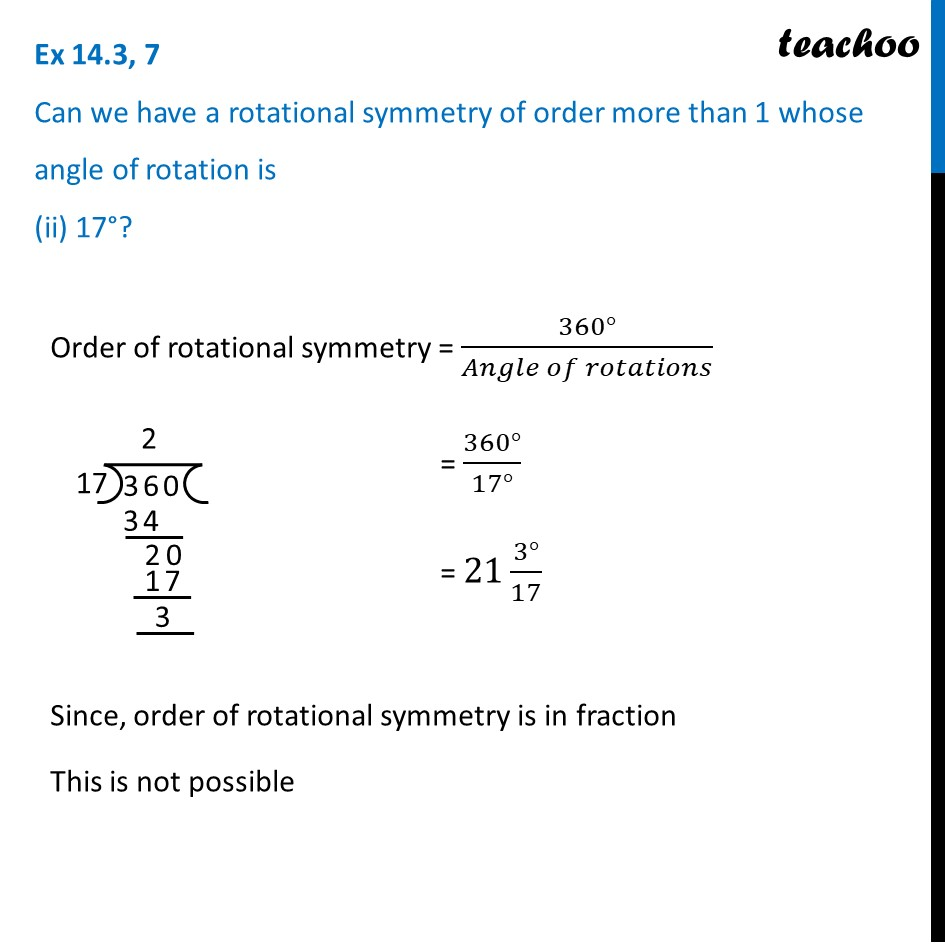Lines and Rotational symmetry (Both)

Chapter 14 Class 7 Symmetry
Concept wiseIntroducing your new favourite teacher - Teachoo Black, at only ₹83 per month

### Transcript

Ex 14.3, 7 Can we have a rotational symmetry of order more than 1 whose angle of rotation is (i) 45°? Order of rotational symmetry = (360°)/(𝐴𝑛𝑔𝑙𝑒 𝑜𝑓 𝑠𝑦𝑚𝑚𝑒𝑡𝑟𝑦) = (360°)/(45°) = (72°)/9 Since, order of rotational symmetry is an integer Hence it is possible Ex 14.3, 7 Can we have a rotational symmetry of order more than 1 whose angle of rotation is (ii) 17°?Order of rotational symmetry = (360°)/(𝐴𝑛𝑔𝑙𝑒 𝑜𝑓 𝑟𝑜𝑡𝑎𝑡𝑖𝑜𝑛𝑠) = (360°)/(17°) = 21 (3°)/17 Since, order of rotational symmetry is in fraction This is not possible